Do you like the article?
Share it with others -

Use new possibilities of MetaTrader 5

# Studying candlestick analysis techniques (part III): Library for pattern operations

22 April 2019, 11:35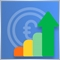5
29 498

### Introduction

We have already considered candlestick analysis techniques: the actuality of the patterns under current market conditions was checked in the first article, and an attempt to expand these research was made in the second article. Using the development evaluation criteria, we studied, tested and compared a wide range of possible pattern combinations. For this purpose, we developed a custom Pattern Analyzer application with a large set of settings for studying patterns. However, the theory and research can only provide information and conclusions. The logical continuation of the task is to use them in real conditions.

### Library structure

Before proceeding to the creation of the library structure, classes and connections, let us define the data which we will use. That is, we need to separate the methods responsible for the input data and the methods which will provide the results. The general library structure will be based on the visual solution developed in the previous articles — the Pattern Analyzer.

Let us start with the application input data which can influence the result when testing the patterns.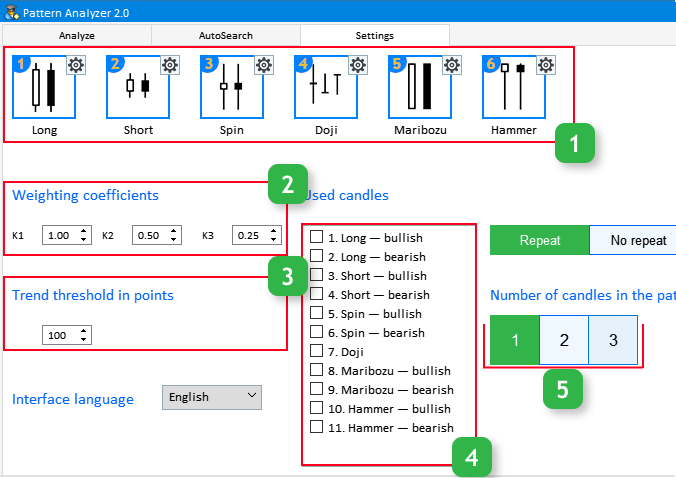Fig.1 Input parameters in the Setting tab.

Block 1. This block features the list of candlestick types which the existing and generated patterns consist of. Each of the types has its settings, which you can view by clicking on the gear icon in the upper right corner of the candlestick visualization page. Candlestick types 1-5 have only one setting, while Hammer has two of them.

Block 2. Weight coefficients. There are three parameters К1, К2, К3 which affect the pattern efficiency evaluation result.

Block 3. Threshold trend value in points.

Block 4. Candlesticks used when testing generated patterns. Here we will need sequence numbers or candlestick indexes. Using this data we will be able to obtain information about any pattern of any size, up to three candlesticks.

Block 5. Number of candlesticks in the pattern. This setting is only applicable for custom patterns.

Then let us view the Analyze tab and input parameters contained therein.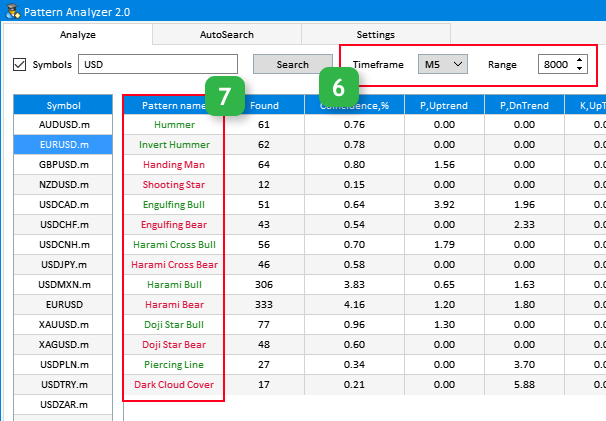Fig.2 Input parameters in the Analyze tab.

Block 6. this block contains settings of the current timeframe and data sample range used for pattern analysis.

Block 7. Names of existing patterns. It also has an input which cannot be edited from the application, but is required for accessing a pattern and obtaining information about it.

Let us enumerate here the data which can be obtained from the pattern analysis. This is needed for the creation of a correct structure of methods in a class.

• Patterns found. The number of found patterns of the specified type.
• Occurrence. The percentage of the number of patterns found from the total sample range.
• Probability of upward or downward movement.
• Efficiency ratios during upward and downward movement for this candlestick pattern.

### Library development

After determining the basic points, let us proceed to library creation. Let us start with the creation of a file with required enumerations Enums.mqh.

```//+------------------------------------------------------------------+
//|                                                        Enums.mqh |
//|                        Copyright 2018, MetaQuotes Software Corp. |
//|                           https://www.mql5.com/en/users/alex2356 |
//+------------------------------------------------------------------+
//+------------------------------------------------------------------+
//| Candlestick type                                                 |
//+------------------------------------------------------------------+
enum TYPE_CANDLESTICK
{
CAND_NONE,           // Undefined
CAND_MARIBOZU,       // Marubozu
CAND_DOJI,           // Doji
CAND_SPIN_TOP,       // Spinning Top
CAND_HAMMER,         // Hammer
CAND_INVERT_HAMMER,  // Inverted Hammer
CAND_LONG,           // Long
CAND_SHORT           // Short
};
//+------------------------------------------------------------------+
//| Pattern type                                                     |
//+------------------------------------------------------------------+
enum TYPE_PATTERN
{
NONE,
HUMMER,
INVERT_HUMMER,
HANDING_MAN,
SHOOTING_STAR,
ENGULFING_BULL,
ENGULFING_BEAR,
HARAMI_BULL,
HARAMI_BEAR,
HARAMI_CROSS_BULL,
HARAMI_CROSS_BEAR,
DOJI_STAR_BULL,
DOJI_STAR_BEAR,
PIERCING_LINE,
DARK_CLOUD_COVER
};
//+------------------------------------------------------------------+
//| Trend type                                                       |
//+------------------------------------------------------------------+
enum TYPE_TREND
{
UPPER,               //Uptrend
DOWN,                //Downtrend
FLAT                 //Flat
};
//+------------------------------------------------------------------+

```

Here we will determine the list of simple candlestick types used, types of existing patterns as well as the type of trend - the data is required for identifying existing patterns on the chart.

After that we will create the Pattern.mqh file. The CPattern class will be created in it, and in its private section we will declare variables for the parameters mentioned in the previous section. Also we need to connect the file with the enumerations.

```//+------------------------------------------------------------------+
//|                                                      Pattern.mqh |
//|                        Copyright 2018, MetaQuotes Software Corp. |
//|                           https://www.mql5.com/en/users/alex2356 |
//+------------------------------------------------------------------+
#include "Enums.mqh"
//+------------------------------------------------------------------+
//|                                                                  |
//+------------------------------------------------------------------+
class CPattern
{
private:
//--- Weights
double            m_k1;
double            m_k2;
double            m_k3;
//--- Threshold trend value in points
int               m_threshold_value;
//--- Long candlestick setup coefficient
double            m_long_coef;
//--- Short candlestick setup coefficient
double            m_short_coef;
//--- Doji candlestick setup coefficient
double            m_doji_coef;
//--- Marubozu candlestick setup coefficient
double            m_maribozu_coef;
//--- Spinning Top candlestick setup coefficient
double            m_spin_coef;
//--- Hammer candlestick setup coefficient
double            m_hummer_coef1;
double            m_hummer_coef2;
//--- Sampling range for the preset patterns
int               m_range_total;
//--- Period to determine the trend
int               m_trend_period;
//--- Found patterns
int               m_found;
//--- Occurrence of patterns
double            m_coincidence;
//--- Probability of upward or downward movement
double            m_probability1;
double            m_probability2;
//--- Efficiency
double            m_efficiency1;
double            m_efficiency2;
//--- Simple candlestick patterns
struct CANDLE_STRUCTURE
{
double            m_open;
double            m_high;
double            m_low;
double            m_close;                      // OHLC
TYPE_TREND        m_trend;                      // Trend
bool              m_bull;                       // Bullish candlestick
double            m_bodysize;                   // Body size
TYPE_CANDLESTICK  m_type;                       // Candlestick type
};
//--- Pattern efficiency evaluation properties
struct RATING_SET
{
int               m_a_uptrend;
int               m_b_uptrend;
int               m_c_uptrend;
int               m_a_dntrend;
int               m_b_dntrend;
int               m_c_dntrend;
};
```

As can be seen from the above code, two structures have been added to our program. The first structure, CANDLE_STRUCTURE is required for determining the candlestick type on the chart. Note that two types of trend enumerations are used in this structure: TYPE_TREND and TYPE_CANDLESTICK from the Enums.mqh file which was considered earlier and which was created for this structure. The second structure, RATING_SET, stores records of price movement estimates after the appearance of the pattern. Please read the first article for more details.

Now consider the part of the public section of the class, which describes the methods for customizing and retrieving the values of input parameters, which are described in the library structure section.

```public:
CPattern(void);
~CPattern(void);
//--- Set and return weight coefficients
void              K1(const double k1)                             { m_k1=k1;                       }
double            K1(void)                                        { return(m_k1);                  }
void              K2(const double k2)                             { m_k2=k2;                       }
double            K2(void)                                        { return(m_k2);                  }
void              K3(const double k3)                             { m_k3=k3;                       }
double            K3(void)                                        { return(m_k3);                  }
//--- Set and return the threshold trend value
void              Threshold(const int threshold)                  { m_threshold_value=threshold;   }
int               Threshold(void)                                 { return(m_threshold_value);     }
//--- Set and return the long candlestick setup coefficient
void              Long_coef(const double long_coef)               { m_long_coef=long_coef;         }
double            Long_coef(void)                                 { return(m_long_coef);           }
//--- Set and return the short candlestick setup coefficient
void              Short_coef(const double short_coef)             { m_short_coef=short_coef;       }
double            Short_coef(void)                                { return(m_short_coef);          }
//--- Set and return the doji candlestick setup coefficient
void              Doji_coef(const double doji_coef)               { m_doji_coef=doji_coef;         }
double            Doji_coef(void)                                 { return(m_doji_coef);           }
//--- Set and return the marubozu candlestick setup coefficient
void              Maribozu_coef(const double maribozu_coef)       { m_maribozu_coef=maribozu_coef; }
double            Maribozu_coef(void)                             { return(m_maribozu_coef);       }
//--- Set and return the Spinning Top candlestick setup coefficient
void              Spin_coef(const double spin_coef)               { m_spin_coef=spin_coef;         }
double            Spin_coef(void)                                 { return(m_spin_coef);           }
//--- Set and return the Hammer candlestick setup coefficient
void              Hummer_coef1(const double hummer_coef1)         { m_hummer_coef1=hummer_coef1;   }
void              Hummer_coef2(const double hummer_coef2)         { m_hummer_coef2=hummer_coef2;   }
double            Hummer_coef1(void)                              { return(m_hummer_coef1);        }
double            Hummer_coef2(void)                              { return(m_hummer_coef2);        }
//--- Set and return the sampling range for preset parameters
void              Range(const int range_total)                    { m_range_total=range_total;     }
int               Range(void)                                     { return(m_range_total);         }
//--- Set and return the number of candlesticks for trend calculation
void              TrendPeriod(const int period)                   { m_trend_period=period;         }
int               TrendPeriod(void)                               { return(m_trend_period);        }
```

In the class constructor, we will describe default parameters, as specified in the application, in the Setting tabs.

```//+------------------------------------------------------------------+
//| Constructor                                                      |
//+------------------------------------------------------------------+
CPattern::CPattern(void) : m_k1(1),
m_k2(0.5),
m_k3(0.25),
m_threshold_value(100),
m_long_coef(1.3),
m_short_coef(0.5),
m_doji_coef(0.04),
m_maribozu_coef(0.01),
m_spin_coef(1),
m_hummer_coef1(0.1),
m_hummer_coef2(2),
m_range_total(8000),
m_trend_period(5)
{
}
```

The second part of the CPattern class public section provides a description of the methods for processing declared input parameters and for obtaining the properties and characteristics of the patterns.

Let us view each of them in detail. It is important to understand the operating algorithm in order to be able to efficiently use them when creating indicators, trading panels or Expert Advisors.

### CandleType

Returns the type of the selected candle from existing patterns listed in TYPE_CANDLESTICK.
`TYPE_CANDLESTICK  CandleType(const string symbol,const ENUM_TIMEFRAMES timeframe,const int shift);`

Parameters

• symbol — Selected symbol for the search
• timeframe  selected timeframe
• shift  Index of the candlestick selected for the analysis starting with.

Return Value

The type of the selected candlestick from the TYPE_CANDLESTICK enumeration.

```//+------------------------------------------------------------------+
//| Candlestick type                                                 |
//+------------------------------------------------------------------+
enum TYPE_CANDLESTICK
{
CAND_NONE,           // Undefined
CAND_MARIBOZU,       // Marubozu
CAND_DOJI,           // Doji
CAND_SPIN_TOP,       // Spinning Top
CAND_HAMMER,         // Hammer
CAND_INVERT_HAMMER,  // Inverted Hammer
CAND_LONG,           // Long
CAND_SHORT           // Short
};
```

Implementation

```//+------------------------------------------------------------------+
//| Returns the type of the selected candlestick                     |
//+------------------------------------------------------------------+
TYPE_CANDLESTICK CPattern::CandleType(const string symbol,const ENUM_TIMEFRAMES timeframe,const int shift)
{
CANDLE_STRUCTURE res;
if(GetCandleType(symbol,timeframe,res,shift))
return(res.m_type);
return(CAND_NONE);
}
//+------------------------------------------------------------------+
//| Candlestick type recognition                                     |
//+------------------------------------------------------------------+
bool CPattern::GetCandleType(const string symbol,const ENUM_TIMEFRAMES timeframe,CANDLE_STRUCTURE &res,const int shift)
{
MqlRates rt[];
int aver_period=m_trend_period;
double aver=0;
SymbolSelect(symbol,true);
int copied=CopyRates(symbol,timeframe,shift,aver_period+1,rt);
//--- Get details of the previous candlestick
if(copied<aver_period)
return(false);
//---
res.m_open=rt[aver_period].open;
res.m_high=rt[aver_period].high;
res.m_low=rt[aver_period].low;
res.m_close=rt[aver_period].close;

//--- Determine the trend direction
for(int i=0;i<aver_period;i++)
aver+=rt[i].close;
aver/=aver_period;

if(aver<res.m_close)
res.m_trend=UPPER;
if(aver>res.m_close)
res.m_trend=DOWN;
if(aver==res.m_close)
res.m_trend=FLAT;
//--- Determine if it is a bullish or a bearish candlestick
res.m_bull=res.m_open<res.m_close;
//--- Get the absolute size of candlestick body
res.m_bodysize=MathAbs(res.m_open-res.m_close);
if(res.m_bull)
{
}
double HL=res.m_high-res.m_low;
//--- Calculate average body size of previous candlesticks
double sum=0;
for(int i=1; i<=aver_period; i++)
sum=sum+MathAbs(rt[i].open-rt[i].close);
sum=sum/aver_period;

//--- Determine the candlestick type
res.m_type=CAND_NONE;
//--- long
if(res.m_bodysize>sum*m_long_coef)
res.m_type=CAND_LONG;
//--- sort
if(res.m_bodysize<sum*m_short_coef)
res.m_type=CAND_SHORT;
//--- doji
if(res.m_bodysize<HL*m_doji_coef)
res.m_type=CAND_DOJI;
//--- marubozu
res.m_type=CAND_MARIBOZU;
//--- hammer
res.m_type=CAND_HAMMER;
//--- invert hammer
res.m_type=CAND_INVERT_HAMMER;
//--- spinning top
res.m_type=CAND_SPIN_TOP;
//---
ArrayFree(rt);
return(true);
}
```

The candlestick recognition method GetCandleType() is used in the public GetCandleType() method. GetCandleType() is a private method. Its arguments include the current symbol, the timeframe and candlestick number, as well as a pointer to the structure. Pattern calculation and identification is performed based on these parameters.

### PatternType

Recognizes the pattern type with the selected candle. It has 5 method overloads because arguments can be either existing patterns from the TYPE_PATTERN enumeration, or generated patterns consisting of one, two or three candlesticks. An argument can also be an array of patterns from the TYPE_PATTERN enumeration.

```   //--- Recognizing the pattern type
bool              PatternType(const string symbol,const ENUM_TIMEFRAMES timeframe,TYPE_PATTERN pattern,int shift);
bool              PatternType(const string symbol,const ENUM_TIMEFRAMES timeframe,int index,const int shift);
bool              PatternType(const string symbol,const ENUM_TIMEFRAMES timeframe,int index1,int index2,const int shift);
bool              PatternType(const string symbol,const ENUM_TIMEFRAMES timeframe,int index1,int index2,int index3,const int shift);
//--- Recognizing the set of patterns
bool              PatternType(const string symbol,const ENUM_TIMEFRAMES timeframe,TYPE_PATTERN &pattern[],int shift);
```

Parameters

• symbol — Symbol selected for search.
• timeframe Selected timeframe.
• pattern,pattern[] Existing pattern type from TYPE_PATTERN.

A pointer to the array of existing patterns from the TYPE_PATTERN list.

```//+------------------------------------------------------------------+
//| Pattern type                                                     |
//+------------------------------------------------------------------+
enum TYPE_PATTERN
{
NONE,
HUMMER,
INVERT_HUMMER,
HANDING_MAN,
SHOOTING_STAR,
ENGULFING_BULL,
ENGULFING_BEAR,
HARAMI_BULL,
HARAMI_BEAR,
HARAMI_CROSS_BULL,
HARAMI_CROSS_BEAR,
DOJI_STAR_BULL,
DOJI_STAR_BEAR,
PIERCING_LINE,
DARK_CLOUD_COVER
};
```
• index,index1,index2,index3  Index of the simple type candlestick (block 4 in Fig.1).
• shift  Index of the candlestick selected for the analysis beginning, starting with 0.

Return Value

A bool value.

Implementation

Since there are 5 types of PatternType method implementation, we will analyze it separately with various arguments. The first of them searches for an existing pattern from the TYPE_PATTERN enumeration. As can be seen below, this is done using the private method CheckPattern.

```//+------------------------------------------------------------------+
//| Recognizing a pre-defined pattern                                |
//+------------------------------------------------------------------+
bool CPattern::PatternType(const string symbol,const ENUM_TIMEFRAMES timeframe,TYPE_PATTERN pattern,const int shift)
{
if(CheckPattern(symbol,timeframe,shift)==pattern)
return(true);
return(false);
}
//+------------------------------------------------------------------+
//| Checks and returns the pattern type                              |
//+------------------------------------------------------------------+
TYPE_PATTERN CPattern::CheckPattern(const string symbol,const ENUM_TIMEFRAMES timeframe,int shift)
{
CANDLE_STRUCTURE cand1,cand2;
TYPE_PATTERN pattern=NONE;
ZeroMemory(cand1);
ZeroMemory(cand2);
GetCandleType(symbol,timeframe,cand2,shift);                                           // Previous candlestick
GetCandleType(symbol,timeframe,cand1,shift-1);                                         // Current candlestick
//--- Inverted Hammer, bullish model
if(cand2.m_trend==DOWN &&                                                              // Checking the trend direction
cand2.m_type==CAND_INVERT_HAMMER)                                                   // Checking the "Inverted Hammer"
pattern=INVERT_HUMMER;
//--- Hanging man, bearish
else if(cand2.m_trend==UPPER && // Checking the trend direction
cand2.m_type==CAND_HAMMER) // Checking "Hammer"
pattern=HANDING_MAN;
//--- Hammer, bullish model
else if(cand2.m_trend==DOWN && // Checking the trend direction
cand2.m_type==CAND_HAMMER) // Checking "Hammer"
pattern=HUMMER;
//--- Shooting Star, bearish model
else if(cand1.m_trend==UPPER && cand2.m_trend==UPPER && // Checking the trend direction
cand2.m_type==CAND_INVERT_HAMMER && cand1.m_close<=cand2.m_open) // Checking "Inverted Hammer"
pattern=SHOOTING_STAR;
//--- Engulfing, bullish model
else if(cand1.m_trend==DOWN && cand1.m_bull && cand2.m_trend==DOWN && !cand2.m_bull && // Checking trend direction and candlestick direction
cand1.m_bodysize>cand2.m_bodysize &&
cand1.m_close>=cand2.m_open && cand1.m_open<cand2.m_close)
pattern=ENGULFING_BULL;
//--- Engulfing, bearish model
else if(cand1.m_trend==UPPER && cand1.m_bull && cand2.m_trend==UPPER && !cand2.m_bull && // Checking trend direction and candlestick direction
cand1.m_bodysize<cand2.m_bodysize &&
cand1.m_close<=cand2.m_open && cand1.m_open>cand2.m_close)
pattern=ENGULFING_BEAR;
//--- Harami Cross, bullish
else if(cand2.m_trend==DOWN && !cand2.m_bull && // Checking trend direction and candlestick direction
(cand2.m_type==CAND_LONG || cand2.m_type==CAND_MARIBOZU) && cand1.m_type==CAND_DOJI && // Checking the "long" first candlestick and the doji candlestick
cand1.m_close<cand2.m_open && cand1.m_open>=cand2.m_close) // Doji is inside the first candlestick body
pattern=HARAMI_CROSS_BULL;
//--- Harami Cross, bearish model
else if(cand2.m_trend==UPPER && cand2.m_bull && // Checking trend direction and candlestick direction
(cand2.m_type==CAND_LONG || cand2.m_type==CAND_MARIBOZU) && cand1.m_type==CAND_DOJI && // Checking the "long" candlestick and the doji candlestick
cand1.m_close>cand2.m_open && cand1.m_open<=cand2.m_close) // Doji is inside the first candlestick body
pattern=HARAMI_CROSS_BEAR;
//--- Harami, bullish
else if(cand1.m_trend==DOWN && cand1.m_bull && !cand2.m_bull && // Checking trend direction and candlestick direction
(cand2.m_type==CAND_LONG || cand2.m_type==CAND_MARIBOZU) && // Checking the "long" first candlestick
cand1.m_type!=CAND_DOJI && cand1.m_bodysize<cand2.m_bodysize && // The second candle is not Doji and first candlestick body is larger than that of the second one
cand1.m_close<cand2.m_open && cand1.m_open>=cand2.m_close) // Second candlestick body is inside the first candlestick body
pattern=HARAMI_BULL;
//--- Harami, bearish
else if(cand1.m_trend==UPPER && !cand1.m_bull && cand2.m_bull && // Checking trend direction and candlestick direction
(cand2.m_type==CAND_LONG || cand2.m_type==CAND_MARIBOZU) && // Checking the "long" first candlestick

cand1.m_type!=CAND_DOJI && cand1.m_bodysize<cand2.m_bodysize && // The second candle is not Doji and first candlestick body is larger than that of the second one
cand1.m_close>cand2.m_open && cand1.m_open<=cand2.m_close) // Second candlestick body is inside the first candlestick body
pattern=HARAMI_BEAR;
//--- Doji Star, bullish
else if(cand1.m_trend==DOWN && !cand2.m_bull && // Checking trend direction and candlestick direction
(cand2.m_type==CAND_LONG || cand2.m_type==CAND_MARIBOZU) && cand1.m_type==CAND_DOJI && // Checking the 1st "long" candlestick and the 2nd doji
cand1.m_close<=cand2.m_open) // Doji Open is lower or equal to 1st candle Close
pattern=DOJI_STAR_BULL;
//--- Doji Star, bearish
else if(cand1.m_trend==UPPER && cand2.m_bull && // Checking trend direction and candlestick direction
(cand2.m_type==CAND_LONG || cand2.m_type==CAND_MARIBOZU) && cand1.m_type==CAND_DOJI && // Checking the 1st "long" candlestick and the 2nd doji
cand1.m_open>=cand2.m_close) //Doji Open price is higher or equal to 1st candle Close
pattern=DOJI_STAR_BEAR;
//--- Piercing, bullish model
else if(cand1.m_trend==DOWN && cand1.m_bull && !cand2.m_bull && // Checking trend direction and candlestick direction
(cand1.m_type==CAND_LONG || cand1.m_type==CAND_MARIBOZU) && (cand2.m_type==CAND_LONG || cand2.m_type==CAND_MARIBOZU) && // Checking the "long" candlestick
cand1.m_close>(cand2.m_close+cand2.m_open)/2 && // 2nd candle Close is above the middle of the 1st candlestick
cand2.m_open>cand1.m_close && cand2.m_close>=cand1.m_open)
pattern=PIERCING_LINE;
//--- Dark Cloud Cover, bearish
else if(cand1.m_trend==UPPER && !cand1.m_bull && cand2.m_bull && // Checking trend direction and candlestick direction
(cand1.m_type==CAND_LONG || cand1.m_type==CAND_MARIBOZU) && (cand2.m_type==CAND_LONG || cand2.m_type==CAND_MARIBOZU) && // Checking the "long" candlestick
cand1.m_close<(cand2.m_close+cand2.m_open)/2 && // Close 2 is below the middle of the 1st candlestick body
cand1.m_close<cand2.m_open && cand2.m_close<=cand1.m_open)
pattern=DARK_CLOUD_COVER;
return(pattern);
}
//+------------------------------------------------------------------+
```

The next type of the PatternType method differs from the previous one in that an array of searched patterns is passed instead of the pattern type argument:

```//+------------------------------------------------------------------+
//| Recognizing an array of patterns                                 |
//+------------------------------------------------------------------+
bool CPattern::PatternType(const string symbol,const ENUM_TIMEFRAMES timeframe,TYPE_PATTERN &pattern[],int shift)
{
for(int i=0;i<ArraySize(pattern);i++)
{
if(CheckPattern(symbol,timeframe,shift)==pattern[i])
return(true);
}
return(false);
}
```

Next, let us consider the implementation of the PatternType method for working with patterns generated from simple candlestick types. There are three types of these patterns: consisting of one, two or three candlesticks. Let us consider them one by one:

```//+------------------------------------------------------------------+
//| Recognizing a pattern by candlestick index                       |
//+------------------------------------------------------------------+
bool CPattern::PatternType(const string symbol,const ENUM_TIMEFRAMES timeframe,int index,int shift)
{
//--- Verify the candlestick index
if(index<0 || index>11)
return(false);
//---
CANDLE_STRUCTURE cand,cur_cand;
RATING_SET ratings;
ZeroMemory(cand);
IndexToPatternType(cand,index);
//--- Get the current candlestick type
GetCandleType(symbol,timeframe,cur_cand,shift);                                // Current candlestick
//---
if(cur_cand.m_type==cand.m_type && cur_cand.m_bull==cand.m_bull)
return(true);
return(false);
}
```

This is implementation of a method searching for a one-candlestick pattern, which is very similar to the CandleType() method, though the type and range of passed arguments is different. Pay attention to the private method IndextoPatternType() which has not been used earlier:

```   //--- Converts the candlestick index to its type
void              IndexToPatternType(CANDLE_STRUCTURE &res,int index);
```

It converts the index of a simple type of candlestick into its type and passes it to the specified structure.

Here is the method for a two-candlestick pattern:

```//+------------------------------------------------------------------+
//| Recognizing a pattern by candlestick index                       |
//+------------------------------------------------------------------+
bool CPattern::PatternType(const string symbol,const ENUM_TIMEFRAMES timeframe,int index1,int index2,int shift)
{
//--- Verify the candlestick index
if(index1<0 || index1>11 || index2<0 || index2>11)
return(false);
//---
CANDLE_STRUCTURE cand1,cand2,cand3,cur_cand,prev_cand;
RATING_SET ratings;
ZeroMemory(cand1);
ZeroMemory(cand2);
IndexToPatternType(cand1,index1);
IndexToPatternType(cand2,index2);
//--- Get the current candlestick type
GetCandleType(symbol,timeframe,prev_cand,shift+1);                             // Previous candlestick
GetCandleType(symbol,timeframe,cur_cand,shift);                                // Current candlestick
//---
if(cur_cand.m_type==cand1.m_type && cur_cand.m_bull==cand1.m_bull &&
prev_cand.m_type==cand2.m_type && prev_cand.m_bull==cand2.m_bull)
return(true);
return(false);
}
```

The code implementation is very similar to the previous one. However, please note that the following feature should be taken into account when selecting candlestick index for analysis: since the pattern consists of two candlesticks, the type candlestick 'index1' will be shifted by 'shift', and for index2 - by 'shift+1'. The same peculiarity concerns the method implementation for three-candlestick patterns:

```//+------------------------------------------------------------------+
//| Recognizing a pattern by candlestick index                       |
//+------------------------------------------------------------------+
bool CPattern::PatternType(const string symbol,const ENUM_TIMEFRAMES timeframe,int index1,int index2,int index3,int shift)
{
CANDLE_STRUCTURE cand1,cand2,cand3,cur_cand,prev_cand,prev_cand2;
RATING_SET ratings;
//---
ZeroMemory(cand1);
ZeroMemory(cand2);
ZeroMemory(cand3);
//---
IndexToPatternType(cand1,index1);
IndexToPatternType(cand2,index2);
IndexToPatternType(cand3,index3);

//--- Get the current candlestick type
GetCandleType(symbol,timeframe,prev_cand2,shift+2);                            // Previous candlestick
GetCandleType(symbol,timeframe,prev_cand,shift+1);                             // Previous candlestick
GetCandleType(symbol,timeframe,cur_cand,shift);                                // Current candlestick
//---
if(cur_cand.m_type==cand1.m_type && cur_cand.m_bull==cand1.m_bull &&
prev_cand.m_type==cand2.m_type && prev_cand.m_bull==cand2.m_bull &&
prev_cand2.m_type==cand3.m_type && prev_cand2.m_bull==cand3.m_bull)
return(true);
return(false);
}
```

### Found

Returns the number of found patterns of the specified type. It has 3 method overloads for existing patterns TYPE_PATTERN, as well as for generated patterns consisting of 1-3 simple candlestick types.

```   //--- Returns the number of found patterns of the specified type
int               Found(const string symbol,const ENUM_TIMEFRAMES timeframe,TYPE_PATTERN pattern);
int               Found(const string symbol,const ENUM_TIMEFRAMES timeframe,int index);
int               Found(const string symbol,const ENUM_TIMEFRAMES timeframe,int index1,int index2);
int               Found(const string symbol,const ENUM_TIMEFRAMES timeframe,int index1,int index2,int index3);
```

Parameters

• symbol  Symbol selected for search.
• timeframe  Selected timeframe.
• patternExisting pattern type from TYPE_PATTERN.
• index,index1,index2,index3 Index of the simple type candlestick (block 4 in Fig.1).

Return Value

The number of found patterns of the specified type.

Implementation

The implementation of this method is quite simple. The main operations related to collecting statistics is performed by the private method PatternStat().

```//+------------------------------------------------------------------+
//| Returns the number of found patterns of the specified type       |
//+------------------------------------------------------------------+
int CPattern::Found(const string symbol,const ENUM_TIMEFRAMES timeframe,TYPE_PATTERN pattern)
{
PatternStat(symbol,timeframe,pattern);
return(m_found);
}
//+------------------------------------------------------------------+
//| Returns the number of found patterns of the specified type       |
//+------------------------------------------------------------------+
int CPattern::Found(const string symbol,const ENUM_TIMEFRAMES timeframe,int index1)
{
PatternStat(symbol,timeframe,index1);
return(m_found);
}
//+------------------------------------------------------------------+
//| Returns the number of found patterns of the specified type       |
//+------------------------------------------------------------------+
int CPattern::Found(const string symbol,const ENUM_TIMEFRAMES timeframe,int index1,int index2)
{
PatternStat(symbol,timeframe,index1,index2);
return(m_found);
}
//+------------------------------------------------------------------+
//| Returns the number of found patterns of the specified type       |
//+------------------------------------------------------------------+
int CPattern::Found(const string symbol,const ENUM_TIMEFRAMES timeframe,int index1,int index2,int index3)
{
PatternStat(symbol,timeframe,index1,index2,index3);
return(m_found);
}
```

The PatternStat() method has two types: for existing and generated patterns. Let us examine them in detail. The first one is intended for patterns from the TYPE_PATTERN enumeration:

```//+------------------------------------------------------------------+
//| Get statistics on the given pattern                              |
//+------------------------------------------------------------------+
CPattern::PatternStat(const string symbol,const ENUM_TIMEFRAMES timeframe,TYPE_PATTERN pattern)
{
//---
int pattern_counter=0;
//---
RATING_SET pattern_coef={0,0,0,0,0,0};
//---
for(int i=m_range_total;i>4;i--)
{
if(CheckPattern(symbol,timeframe,i)==pattern)
{
pattern_counter++;
if(pattern==HUMMER || pattern==INVERT_HUMMER || pattern==HANDING_MAN)
GetCategory(symbol,timeframe,pattern_coef,i-3);
else
GetCategory(symbol,timeframe,pattern_coef,i-4);
}
}
//---
CoefCalculation(pattern_coef,pattern_counter);
}
```

The second one is used for generated patterns consisting of indexes of simple candlestick types.

```//+------------------------------------------------------------------+
//| Get statistics on the given pattern                              |
//+------------------------------------------------------------------+
void CPattern::PatternStat(const string symbol,const ENUM_TIMEFRAMES timeframe,int index1,int index2=0,int index3=0)
{
CANDLE_STRUCTURE cand1,cand2,cand3,cur_cand,prev_cand,prev_cand2;
RATING_SET rating={0,0,0,0,0,0};
int pattern_total=0,pattern_size=1;
//---
ZeroMemory(cand1);
ZeroMemory(cand2);
ZeroMemory(cand3);
ZeroMemory(cur_cand);
ZeroMemory(prev_cand);
ZeroMemory(prev_cand2);
//---
if(index2>0)
pattern_size=2;
if(index3>0)
pattern_size=3;
//---
if(pattern_size==1)
IndexToPatternType(cand1,index1);
else if(pattern_size==2)
{
IndexToPatternType(cand1,index1);
IndexToPatternType(cand2,index2);
}
else if(pattern_size==3)
{
IndexToPatternType(cand1,index1);
IndexToPatternType(cand2,index2);
IndexToPatternType(cand3,index3);
}
//---
for(int i=m_range_total;i>5;i--)
{
if(pattern_size==1)
{
//--- Get the current candlestick type
GetCandleType(symbol,timeframe,cur_cand,i);                                       // Current candlestick
//---
if(cur_cand.m_type==cand1.m_type && cur_cand.m_bull==cand1.m_bull)
{
pattern_total++;
GetCategory(symbol,timeframe,rating,i-3);
}
}
else if(pattern_size==2)
{
//--- Get the current candlestick type
GetCandleType(symbol,timeframe,prev_cand,i);                                        // Previous candlestick
GetCandleType(symbol,timeframe,cur_cand,i-1);                                       // Current candlestick
//---

if(cur_cand.m_type==cand1.m_type && cur_cand.m_bull==cand1.m_bull &&
prev_cand.m_type==cand2.m_type && prev_cand.m_bull==cand2.m_bull)
{
pattern_total++;
GetCategory(symbol,timeframe,rating,i-4);
}
}
else if(pattern_size==3)
{
//--- Get the current candlestick type
GetCandleType(symbol,timeframe,prev_cand2,i);                                       // Previous candlestick
GetCandleType(symbol,timeframe,prev_cand,i-1);                                      // Previous candlestick
GetCandleType(symbol,timeframe,cur_cand,i-2);                                       // Current candlestick
//---
if(cur_cand.m_type==cand1.m_type && cur_cand.m_bull==cand1.m_bull &&
prev_cand.m_type==cand2.m_type && prev_cand.m_bull==cand2.m_bull &&
prev_cand2.m_type==cand3.m_type && prev_cand2.m_bull==cand3.m_bull)
{
pattern_total++;
GetCategory(symbol,timeframe,rating,i-5);
}
}
}
//---
CoefCalculation(rating,pattern_total);
}
```

These two method implementations contain one new method. This is the private method CoefCalculation():

```   //--- Calculates coefficients
bool              CoefCalculation(RATING_SET &rate,int found);
```

It processes all the results obtained when searching and testing patterns. Its arguments contain a pointer to the RATING_SET structure which is responsible for the collection of data concerning pattern efficiency testing results and the number of found patterns. All other parameters and properties of analyzed patterns are calculated in the CoefCalculation() method.

```//+------------------------------------------------------------------+
//| Calculating efficiency assessment coefficients                   |
//+------------------------------------------------------------------+
bool CPattern::CoefCalculation(RATING_SET &rate,int found)
{
int sum1=0,sum2=0;
sum1=rate.m_a_uptrend+rate.m_b_uptrend+rate.m_c_uptrend;
sum2=rate.m_a_dntrend+rate.m_b_dntrend+rate.m_c_dntrend;
//---
m_probability1=(found>0)?NormalizeDouble((double)sum1/found*100,2):0;
m_probability2=(found>0)?NormalizeDouble((double)sum2/found*100,2):0;
m_efficiency1=(found>0)?NormalizeDouble((m_k1*rate.m_a_uptrend+m_k2*rate.m_b_uptrend+m_k3*rate.m_c_uptrend)/found,3):0;
m_efficiency2=(found>0)?NormalizeDouble((m_k1*rate.m_a_dntrend+m_k2*rate.m_b_dntrend+m_k3*rate.m_c_dntrend)/found,3):0;
m_found=found;
m_coincidence=((double)found/m_range_total*100);
return(true);
}
```

### Coincidence

Returns the frequency of pattern occurrence. It has 3 method overloads for existing patterns TYPE_PATTERN, as well as for generated patterns consisting of 1-3 simple candlestick types.

```   //--- Returns the frequency of the pattern occurrence
double            Coincidence(const string symbol,const ENUM_TIMEFRAMES timeframe,TYPE_PATTERN pattern);
double            Coincidence(const string symbol,const ENUM_TIMEFRAMES timeframe,int index);
double            Coincidence(const string symbol,const ENUM_TIMEFRAMES timeframe,int index1,int index2);
double            Coincidence(const string symbol,const ENUM_TIMEFRAMES timeframe,int index1,int index2,int index3);
```

Parameters

• symbolSymbol selected for search.
• timeframeSelected timeframe.
• patternExisting pattern type from TYPE_PATTERN.
• index,index1,index2,index3Index of the simple type candlestick (block 4 in Fig.1).

Return Value

The frequency of pattern occurrence in percent.

Implementation

Similar to the Found() method. The only difference is that it returns the value of the m_coincidence variable.

```//+------------------------------------------------------------------+
//| Returns the frequency of the pattern occurrence                  |
//+------------------------------------------------------------------+
double CPattern::Coincidence(const string symbol,const ENUM_TIMEFRAMES timeframe,TYPE_PATTERN pattern)
{
PatternStat(symbol,timeframe,pattern);
return(m_coincidence);
}
//+------------------------------------------------------------------+
//| Returns the frequency of the pattern occurrence                  |
//+------------------------------------------------------------------+
double CPattern::Coincidence(const string symbol,const ENUM_TIMEFRAMES timeframe,int index1)
{
PatternStat(symbol,timeframe,index1);
return(m_coincidence);
}
//+------------------------------------------------------------------+
//| Returns the frequency of the pattern occurrence                  |
//+------------------------------------------------------------------+
double CPattern::Coincidence(const string symbol,const ENUM_TIMEFRAMES timeframe,int index1,int index2)
{
PatternStat(symbol,timeframe,index1,index2);
return(m_coincidence);
}
//+------------------------------------------------------------------+
//| Returns the frequency of the pattern occurrence                  |
//+------------------------------------------------------------------+
double CPattern::Coincidence(const string symbol,const ENUM_TIMEFRAMES timeframe,int index1,int index2,int index3)
{
PatternStat(symbol,timeframe,index1,index2,index3);
return(m_coincidence);
}
```

### Probability

Returns the percentage probability of movement after the given pattern. It has 3 method overloads for existing patterns TYPE_PATTERN, as well as for generated patterns consisting of 1-3 simple candlestick types.

```   //--- Returns the probability of movement after the given pattern
double            Probability(const string symbol,const ENUM_TIMEFRAMES timeframe,TYPE_PATTERN pattern,TYPE_TREND trend);
double            Probability(const string symbol,const ENUM_TIMEFRAMES timeframe,int index,TYPE_TREND trend);
double            Probability(const string symbol,const ENUM_TIMEFRAMES timeframe,int index1,int index2,TYPE_TREND trend);
double            Probability(const string symbol,const ENUM_TIMEFRAMES timeframe,int index1,int index2,int index3,TYPE_TREND trend);
```

Parameters

• symbolSymbol selected for search.
• timeframeSelected timeframe.
• patternExisting pattern type from TYPE_PATTERN.
• index,index1,index2,index 3Index of the simple type candlestick (block 4 in Fig.1).

• trendTYPE_TREND
```//+------------------------------------------------------------------+
//| Trend type                                                       |
//+------------------------------------------------------------------+
enum TYPE_TREND
{
UPPER,               //Uptrend
DOWN,                //Downtrend
FLAT                 //Flat
};
//+------------------------------------------------------------------+
```

Return Value

The percentage probability of movement after the given pattern.

Implementation

Similar to the Found() method. The only difference is that it returns the value of the m_probability1 or m_probability2 variable depending on the selected trend type.

```//+------------------------------------------------------------------+
//| Returns the probability of movement after the given pattern      |
//+------------------------------------------------------------------+
double CPattern::Probability(const string symbol,const ENUM_TIMEFRAMES timeframe,TYPE_PATTERN pattern,TYPE_TREND trend)
{
PatternStat(symbol,timeframe,pattern);
if(trend==UPPER)
return(m_probability1);
if(trend==DOWN)
return(m_probability2);
return(0);
}
//+------------------------------------------------------------------+
//| Returns the probability of movement after the given pattern      |
//+------------------------------------------------------------------+
double CPattern::Probability(const string symbol,const ENUM_TIMEFRAMES timeframe,int index1,TYPE_TREND trend)
{
PatternStat(symbol,timeframe,index1);
if(trend==UPPER)
return(m_probability1);
if(trend==DOWN)
return(m_probability2);
return(0);
}
//+------------------------------------------------------------------+
//| Returns the probability of movement after the given pattern      |
//+------------------------------------------------------------------+
double CPattern::Probability(const string symbol,const ENUM_TIMEFRAMES timeframe,int index1,int index2,TYPE_TREND trend)
{
PatternStat(symbol,timeframe,index1,index2);
if(trend==UPPER)
return(m_probability1);
if(trend==DOWN)
return(m_probability2);
return(0);
}
//+------------------------------------------------------------------+
//| Returns the probability of movement after the given pattern      |
//+------------------------------------------------------------------+
double CPattern::Probability(const string symbol,const ENUM_TIMEFRAMES timeframe,int index1,int index2,int index3,TYPE_TREND trend)
{
PatternStat(symbol,timeframe,index1,index2,index3);
if(trend==UPPER)
return(m_probability1);
if(trend==DOWN)
return(m_probability2);
return(0);
}
```

### Efficiency

Returns the pattern efficiency coefficient. It has 3 method overloads for existing patterns TYPE_PATTERN, as well as for generated patterns consisting of 1-3 simple candlestick types.

```   //--- Returns the pattern efficiency coefficient
double            Efficiency(const string symbol,const ENUM_TIMEFRAMES timeframe,TYPE_PATTERN pattern,TYPE_TREND trend);
double            Efficiency(const string symbol,const ENUM_TIMEFRAMES timeframe,int index,TYPE_TREND trend);
double            Efficiency(const string symbol,const ENUM_TIMEFRAMES timeframe,int index1,int index2,TYPE_TREND trend);
double            Efficiency(const string symbol,const ENUM_TIMEFRAMES timeframe,int index1,int index2,int index3,TYPE_TREND trend);
```

Parameters

• symbolSymbol selected for search.
• timeframeSelected timeframe.
• patternExisting pattern type from TYPE_PATTERN.
• index,index1,index2,index3Index of the simple type candlestick (block 4 in Fig.1).
• trendtrend type, TYPE_TREND
```//+------------------------------------------------------------------+
//| Trend type                                                       |
//+------------------------------------------------------------------+
enum TYPE_TREND
{
UPPER,               //Uptrend
DOWN,                //Downtrend
FLAT                 //Flat
};
//+------------------------------------------------------------------+
```

Return Value

The pattern efficiency coefficient.

Implementation

Similar to the Found() method. The only difference is that it returns the value of the m_efficiency1 or m_efficiency2 variable depending on the selected trend type.

```//+------------------------------------------------------------------+
//| Returns the probability of movement after the given pattern      |
//+------------------------------------------------------------------+
double CPattern::Efficiency(const string symbol,const ENUM_TIMEFRAMES timeframe,TYPE_PATTERN pattern,TYPE_TREND trend)
{
PatternStat(symbol,timeframe,pattern);
if(trend==UPPER)
return(m_efficiency1);
if(trend==DOWN)
return(m_efficiency2);
return(0);
}
//+------------------------------------------------------------------+
//| Returns the probability of movement after the given pattern      |
//+------------------------------------------------------------------+
double CPattern::Efficiency(const string symbol,const ENUM_TIMEFRAMES timeframe,int index1,TYPE_TREND trend)
{
PatternStat(symbol,timeframe,index1);
if(trend==UPPER)
return(m_efficiency1);
if(trend==DOWN)
return(m_efficiency2);
return(0);
}
//+------------------------------------------------------------------+
//| Returns the probability of movement after the given pattern      |
//+------------------------------------------------------------------+
double CPattern::Efficiency(const string symbol,const ENUM_TIMEFRAMES timeframe,int index1,int index2,TYPE_TREND trend)
{
PatternStat(symbol,timeframe,index1,index2);
if(trend==UPPER)
return(m_efficiency1);
if(trend==DOWN)
return(m_efficiency2);
return(0);
}
//+------------------------------------------------------------------+
//| Returns the probability of movement after the given pattern      |
//+------------------------------------------------------------------+
double CPattern::Efficiency(const string symbol,const ENUM_TIMEFRAMES timeframe,int index1,int index2,int index3,TYPE_TREND trend)
{
PatternStat(symbol,timeframe,index1,index2,index3);
if(trend==UPPER)
return(m_efficiency1);
if(trend==DOWN)
return(m_efficiency2);
return(0);
}
```

### Practical use

Having considered tools for working with patterns, let us implement two indicators and an Expert Advisor to demonstrate library use examples.

### CandleDetector

Let us start with the indicator which shows on a chart a candlestick of the selected type from the TYPE_CANDLESTICK enumeration. Create the Pattern folder in the Indicators folder. In this folder, create the CandleDetector.mq5 file in which the indicator will be created. Let us connect the Pattern.mqh library for pattern operations and set initial properties of the future indicator:

```//+------------------------------------------------------------------+
//|                                               CandleDetector.mq5 |
//|                        Copyright 2019, MetaQuotes Software Corp. |
//|                           https://www.mql5.com/en/users/alex2356 |
//+------------------------------------------------------------------+
#property version   "1.00"
#property indicator_chart_window
#property indicator_buffers 1
#property indicator_plots   1
#include <Pattern/Pattern.mqh>
//+----------------------------------------------+
//|  Indicator drawing parameters                |
//+----------------------------------------------+
//---- Drawing the indicator as a label
#property indicator_type1   DRAW_ARROW
//---- Indicator line width
#property indicator_width1  1
```

The next step is to determine key settings which will affect the display of this or that candlestick type.

```//+----------------------------------------------+
//| Indicator input parameters                   |
//+----------------------------------------------+
input TYPE_CANDLESTICK  CandleType=1;                 // Candlestick type
input color             LabelColor=clrCrimson;
input double            LongCoef=1.3;
input double            ShortCoef=0.5;
input double            DojiCoef=0.04;
input double            MaribozuCoef=0.01;
input double            SpinCoef=1;
input double            HummerCoef1=0.1;
input double            HummerCoef2=2;
input int               TrendPeriod=5;
```

Next, in the initialization, configure the indicator appearance and determine all the values from input parameters for search.

```//+------------------------------------------------------------------+
//| Custom indicator initialization function                         |
//+------------------------------------------------------------------+
int OnInit()
{
//---- Initialize variables of data calculation start
min_rates_total=TrendPeriod+1;
//---- Define the accuracy of indicator values to be displayed
IndicatorSetInteger(INDICATOR_DIGITS,_Digits);

//---- Set the dynamic array Signal[] as an indicator buffer
SetIndexBuffer(0,Signal,INDICATOR_DATA);
//---- Shift indicator 1 drawing start
PlotIndexSetInteger(0,PLOT_DRAW_BEGIN,min_rates_total);
//---- Set indexing of elements in buffers as in timeseries
ArraySetAsSeries(Signal,true);
//---- Set indicator values which will not be visible on the chart
PlotIndexSetDouble(0,PLOT_EMPTY_VALUE,EMPTY_VALUE);
//---- Indicator symbol
PlotIndexSetInteger(0,PLOT_ARROW,108);
//---
PlotIndexSetInteger(0,PLOT_LINE_COLOR,LabelColor);
//----
Pat.Long_coef(LongCoef);
Pat.Short_coef(ShortCoef);
Pat.Doji_coef(DojiCoef);
Pat.Maribozu_coef(MaribozuCoef);
Pat.Spin_coef(SpinCoef);
Pat.Hummer_coef1(HummerCoef1);
Pat.Hummer_coef2(HummerCoef2);
Pat.TrendPeriod(TrendPeriod);
return(INIT_SUCCEEDED);
}
```

Pay attention to the CandleType() method — it is used to search for the selected candlestick type on the chart.

```//+------------------------------------------------------------------+
//| Custom indicator iteration function                              |
//+------------------------------------------------------------------+
int OnCalculate(const int rates_total,
const int prev_calculated,
const datetime &time[],
const double &open[],
const double &high[],
const double &low[],
const double &close[],
const long &tick_volume[],
const long &volume[],
{
//---- Check if there are enough bars for calculation
if(rates_total<min_rates_total)
return(0);
//---- Declare local variables
int limit,bar;
//---- Set indexing of elements in arrays as in timeseries
ArraySetAsSeries(low,true);
//---- Calculate the 'first' starting number for the bars recalculation cycle
if(prev_calculated>rates_total || prev_calculated<=0)          // Check the first start of indicator calculation
limit=rates_total-min_rates_total;                          // Starting index for calculating all bars
else
limit=rates_total-prev_calculated;                          // Starting index for calculating new bars
//---- Main indicator calculation loop
for(bar=limit; bar>=0; bar--)
{
Signal[bar]=0.0;
if(Pat.CandleType(Symbol(),PERIOD_CURRENT,bar)==CandleType)
Signal[bar]=low[bar]-200*_Point;
}
return(rates_total);
}
//+------------------------------------------------------------------+
```

The indicator operation example is shown in Fig.3 (Search for a Long type candlestick).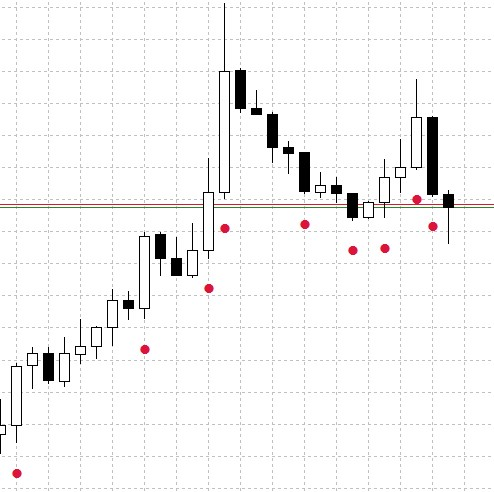Fig.3 The CandleDetector indicator operation example.

### PatternDetector

The second indicator will search for a specified pattern from the TYPE_PATTERN enumeration. The implementation is very similar to the previous one. This code differs in one input parameter and in the method used in the calculation part.

```//+----------------------------------------------+
//| Indicator input parameters                   |
//+----------------------------------------------+
input TYPE_PATTERN      PatternType=1;                // Pattern type
input color             LabelColor=clrCrimson;
input double            LongCoef=1.3;
input double            ShortCoef=0.5;
input double            DojiCoef=0.04;
input double            MaribozuCoef=0.01;
input double            SpinCoef=1;
input double            HummerCoef1=0.1;
input double            HummerCoef2=2;
input int               TrendPeriod=5;
//---
CPattern Pat;
double Signal[];
//---- Declare integer variables for the data calculation start
int min_rates_total;
//+------------------------------------------------------------------+
//| Custom indicator initialization function                         |
//+------------------------------------------------------------------+
void OnInit()
{
//---- Initialize variables of data calculation start
min_rates_total=TrendPeriod+2;
//---- Define the accuracy of indicator values to be displayed
IndicatorSetInteger(INDICATOR_DIGITS,_Digits);

//---- Set SignUp[] dynamic array as an indicator buffer
SetIndexBuffer(0,Signal,INDICATOR_DATA);
//---- Shift indicator 1 drawing start
PlotIndexSetInteger(0,PLOT_DRAW_BEGIN,min_rates_total);
//---- Set indexing of elements in buffers as in timeseries
ArraySetAsSeries(Signal,true);
//---- Set indicator values which will not be visible on the chart
PlotIndexSetDouble(0,PLOT_EMPTY_VALUE,EMPTY_VALUE);
//---- Indicator symbol
PlotIndexSetInteger(0,PLOT_ARROW,108);
//---
PlotIndexSetInteger(0,PLOT_LINE_COLOR,LabelColor);
//----
Pat.Long_coef(LongCoef);
Pat.Short_coef(ShortCoef);
Pat.Doji_coef(DojiCoef);
Pat.Maribozu_coef(MaribozuCoef);
Pat.Spin_coef(SpinCoef);
Pat.Hummer_coef1(HummerCoef1);
Pat.Hummer_coef2(HummerCoef2);
Pat.TrendPeriod(TrendPeriod);
}
//+------------------------------------------------------------------+
//| Custom indicator iteration function                              |
//+------------------------------------------------------------------+
int OnCalculate(const int rates_total,
const int prev_calculated,
const datetime &time[],
const double &open[],
const double &high[],
const double &low[],
const double &close[],
const long &tick_volume[],
const long &volume[],
{
//---- Check if there are enough bars for calculation
if(rates_total<min_rates_total)
return(0);
//---- Declare local variables
int limit,bar;
//---- Set indexing of elements in arrays as in timeseries
ArraySetAsSeries(low,true);
//---- Calculate the 'first' starting number for the bars recalculation cycle
if(prev_calculated>rates_total || prev_calculated<=0)       // Check the first start of indicator calculation
limit=rates_total-min_rates_total;                       // Starting index for calculating all bars
else
limit=rates_total-prev_calculated;                       // Starting index for calculating new bars
//---- Main indicator calculation loop
for(bar=limit; bar>0; bar--)
{
Signal[bar]=0.0;
if(Pat.PatternType(_Symbol,_Period,PatternType,bar))
Signal[bar]=low[bar]-200*_Point;
}
return(rates_total);
}
//+------------------------------------------------------------------+
```

PatternDetector operation results are shown in Fig.4 (search for the Engulfing - bullish pattern).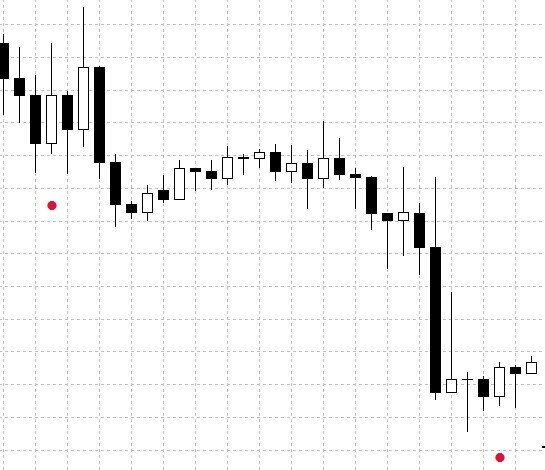Fig.4 The PatternDetector indicator operation example.

Now let us create an Expert Advisor which can find patterns on the chart and open positions depending on the selected pattern. Individual pattern types can be used for each deal type. An additional mode will allow the choice between existing patterns and patterns generated using simple candlestick types.

Let us create the Pattern folder in the Experts folder and add the PatternExpert.mq5, in which the EA code will be written. At the first stage, connect the Pattern.mqh library for working with patterns and the Trade.mqh trade operations library. Declare class instances and introduce the PATTERN_MODE enumeration which enables switching between existing and generated patterns.

```//+------------------------------------------------------------------+
//|                                                PatternExpert.mq5 |
//|                        Copyright 2019, MetaQuotes Software Corp. |
//|                           https://www.mql5.com/en/users/alex2356 |
//+------------------------------------------------------------------+
#property version   "1.00"
#include <Pattern/Pattern.mqh>
CPattern Pat;
//+------------------------------------------------------------------+
//| Pattern search mode                                              |
//+------------------------------------------------------------------+
enum PATTERN_MODE
{
EXISTING,
GENERATED
};
```

Now define the Expert Advisor input parameters. EA parameters are provided in the first block:

```//+------------------------------------------------------------------+
//| Expert Advisor input parameters                                  |
//+------------------------------------------------------------------+
input    string               Inp_EaComment="Pattern Strategy";         // EA Comment
input    double               Inp_Lot=0.01;                             // Lot
input    MarginMode           Inp_MMode=LOT;                            // Money Management

//--- EA parameters
input    string               Inp_Str_label="===EA parameters===";      // Label
input    int                  Inp_MagicNum=1111;                        // Magic number
input    int                  Inp_StopLoss=40;                          // Stop Loss(points)
input    int                  Inp_TakeProfit=30;                        // Take Profit(points)
```

The second part contains settings and trading parameters.

```//--- Trading parameters
input ENUM_TIMEFRAMES         Timeframe=PERIOD_CURRENT;                 // Current Timeframe
input PATTERN_MODE            PatternMode=0;                            // Pattern Mode
input TYPE_PATTERN            SellPatternType=ENGULFING_BEAR;           // Sell Pattern Type
input uint                    SellIndex1=1;                             // SellIndex of simple candle1
input uint                    SellIndex2=0;                             // SellIndex of simple candle2
input uint                    SellIndex3=0;                             // SellIndex of simple candle3
input double                  LongCoef=1.3;                             // Long candle coef
input double                  ShortCoef=0.5;                            // Short candle coef
input double                  DojiCoef=0.04;                            // Doji candle coef
input double                  MaribozuCoef=0.01;                        // Maribozu candle coef
input double                  SpinCoef=1;                               // Spin candle coef
input double                  HummerCoef1=0.1;                          // Hummer candle coef1
input double                  HummerCoef2=2;                            // Hummer candle coef2
input int                     TrendPeriod=5;                            // Trend Period
```

Let's consider some of these parameters in more detail:

• Current Timeframe — timeframe selected for operations. Enables timeframe selection during optimization. The current chart timeframe is selected by default.
• Pattern Mode — pattern selection mode. EXISTING — existing patterns; two settings Buy Pattern Type and Sell Pattern Type are applicable in this mode. GENERATED - generated patterns. In this mode, Buy/Sell Pattern Type settings are ignored and BuyIndex1-3 and SellIndex1-3 are used instead.
• Buy Pattern Type/ Sell Pattern Type — select patterns for opening appropriate trading operations.
• BuyIndex1-3/SellIndex1-3 — select a pattern generated of simple candlestick types (Fig.1 Block 4), upon the emergence of which a long/short position will be opened.

Other parameters are similar to the above considered indicators. In addition to checks, set in the initialization blocks the Trend Period value which affects on-chart detection of patterns.

```//+------------------------------------------------------------------+
//| Expert initialization function                                   |
//+------------------------------------------------------------------+
int OnInit()
{
//--- Checking connection to the trade server
if(!TerminalInfoInteger(TERMINAL_CONNECTED))
{
Print(Inp_EaComment,": No Connection!");
return(INIT_FAILED);
}
{
return(INIT_FAILED);
}
//---
Pat.TrendPeriod(TrendPeriod);
//---
return(INIT_SUCCEEDED);
}
```

The calculation part is easy to understand. Let us consider buy and sell signal search functions.

```//+------------------------------------------------------------------+
//| Expert tick function                                             |
//+------------------------------------------------------------------+
void OnTick()
{
{
//--- Opening an order if there is a buy signal
//--- Opening an order if there is a sell signal
if(SellSignal())
}
}
```

These functions are similar and thus let us consider one of them BuySignal(), which searches for a buy signal.

```//+------------------------------------------------------------------+
//+------------------------------------------------------------------+
{
if(PatternMode==0)
{
return(false);
return(true);
}
else if(PatternMode==1)
{
{
return(true);
}
{
return(true);
}
{
return(true);
}
}
return(false);
}
```

The function contains a check of the currently selected mode, existing patterns or generated patterns. Input parameters are selected accordingly: TYPE_PATTERN or a set of indexes of the generated pattern.

Let us test and optimize the resulting Expert Advisor in two modes: with existing patterns from the TYPE_PATTERN enumeration and with generated patterns consisting of simple candlestick types shown in Fig.1 Block 4.

The Expert Advisor will be tested with the following parameters:

• Interval: for the Uptrend mode 01.01.2018 — 15.03.2018.
• Currency pair: EURUSD.
• Trading mode: No delay. These are not high-frequency trading strategies, so the effect of delays is very small.
• Testing: 1 Minute OHLC.
• Initial deposit: 1000 USD.
• Leverage: 1:500.
• Server: MetaQuotes-Demo.
• Quotes: 5-digit.

Generated Patterns mode.

Let us determine parameters to be tested and optimized.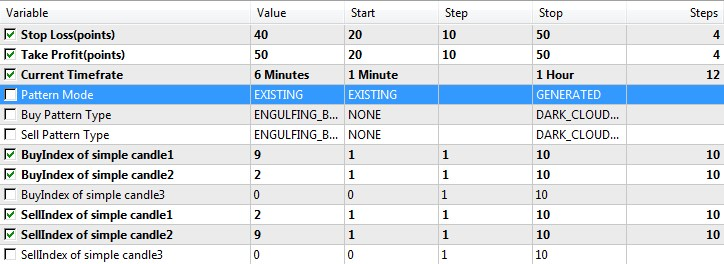Fig.5 Set of parameters for optimization in the Generated Patterns mode.

Fig.5 shows testing and optimization conditions. The best parameters obtained as a result of testing are shown in the second Value column. The back testing results and chart are shown in Figure 6 below.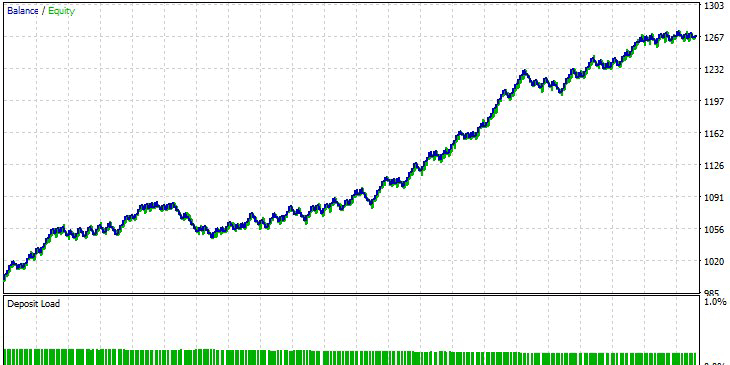Fig.6 Best parameter testing results in the Generated Patterns mode.

Existing Patterns mode.

Set parameters for testing and optimization.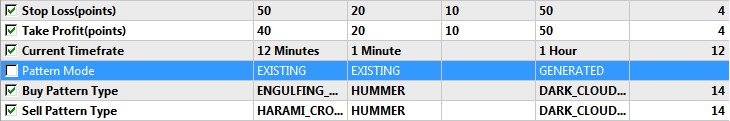Fig.7 Set of parameters for optimization in the Existing Patterns mode.

Again, parameters of the best optimization result are shown in the second column. Now let us perform a single test. The results are shown below, in Figure 8.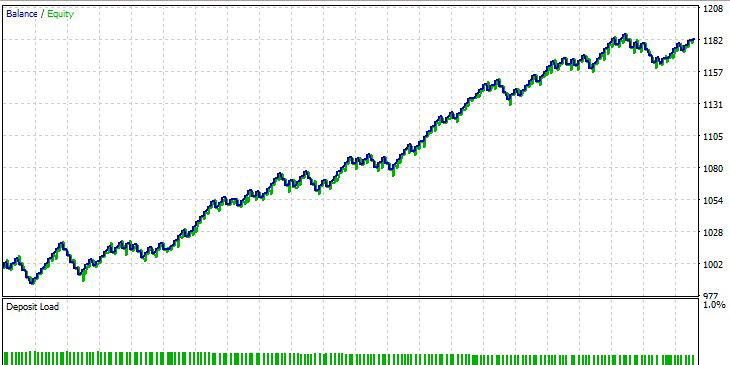Fig.8 Best parameter testing results in the Existing Patterns mode.

### Conclusions

The archive attached below contains all described files properly arranged into folders. For a correct operation, you should save the MQL5 folder to the terminal's root directory. To find this root folder in which the MQL5 folder is located, press Ctrl+Shift+D in MetaTrader 5 or use the context menu as shown in Figure 9.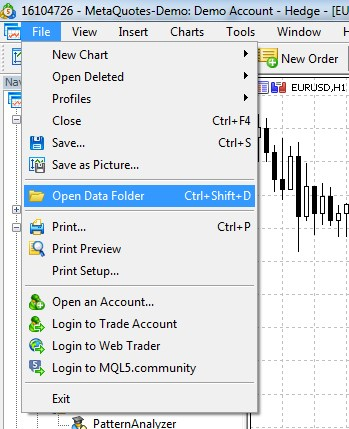Fig.9 How to find the MQL5 folder in the MetaTrader5 root directory.

Programs used in the article

#
Name
Type
Description
1
Pattern.mqh Library  Library for working with patterns
2 CandleDetector.mq5 Indicator  Candlestick searching indicator
3 PatternDetector.mq5 Indicator  Pattern searching indicator

Translated from Russian by MetaQuotes Software Corp.
Original article: https://www.mql5.com/ru/articles/5751

Attached files |
MQL5.zip (452.51 KB)
Last comments | Go to discussion (5)| 26 Apr 2019 at 09:48

Great work, Alexander..

I am a newbie in mql5 programming. I've tried to compile the PatternAnalyzer.mq5 but it ended up with 2 error found on 'method' which took place in Menultem.mqh.

First error on 'method' was "undeclared identifier", and the second was "some operator expected".

I do appreciate your work. Thank you..| 26 Apr 2019 at 10:42
bqFX:

Great work, Alexander..

I am a newbie in mql5 programming. I've tried to compile the PatternAnalyzer.mq5 but it ended up with 2 error found on 'method' which took place in Menultem.mqh.

First error on 'method' was "undeclared identifier", and the second was "some operator expected".

I do appreciate your work. Thank you..

Incorrectly installed| 30 Apr 2019 at 02:02

Hi Alexander! I am grateful for your voluntary work. Please on install the MQL5 file I noticed there are other files in the

folder like include, image expert and indicator. My question is should we just copy MQL5 folder to the root directory

or should we copy out expert to expert and indicator to indicator etc..

Thanks| 5 May 2019 at 06:49

is this transferrable to mq4? Will the libraries work the same in mq4/mt4?

I love the article too. Thanks for your contribution!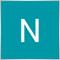| 24 Jul 2019 at 07:25

Hi Alexander, i am grateful if you give us a real data backtesting because i was tested your's ea and it doesn't working like you post..

Here the screenshot of it..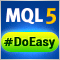Library for easy and quick development of MetaTrader programs (part I). Concept, data management and first results

While analyzing a huge number of trading strategies, orders for development of applications for MetaTrader 5 and MetaTrader 4 terminals and various MetaTrader websites, I came to the conclusion that all this diversity is based mostly on the same elementary functions, actions and values appearing regularly in different programs. This resulted in DoEasy cross-platform library for easy and quick development of МetaТrader 5 and МetaТrader 4 applications.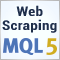Scraping bond yield data from the web

Automate the collection of interest rate data to improve the performance of an Expert Advisor.Library for easy and quick development of MetaTrader programs (part II). Collection of historical orders and deals

In the first part, we started creating a large cross-platform library simplifying the development of programs for MetaTrader 5 and MetaTrader 4 platforms. We created the COrder abstract object which is a base object for storing data on history orders and deals, as well as on market orders and positions. Now we will develop all the necessary objects for storing account history data in collections.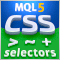Extracting structured data from HTML pages using CSS selectors

The article provides a description of a universal method for analyzing and converting data from HTML documents based on CSS selectors. Trading reports, tester reports, your favorite economic calendars, public signals, account monitoring and additional online quote sources will become available straight from MQL.